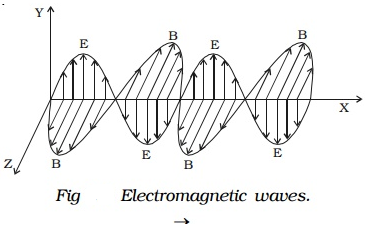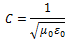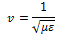Electromagnetic waves with the wavelength of the order of a few meters were first produced and detected in the laboratory by Hertz in 1887. He thus verified a basic prediction of Maxwell’s equations.

According to Maxwell, an accelerated charge is a source of electromagnetic radiation.

In an electromagnetic wave, electric and magnetic field vectors are at right angles to each other and both are at right angles to the direction of propagation. They possess the wave character and propagate through free space without any material medium. These waves are transverse in nature.In this fig. shows the variation of an electric field along Y direction and magnetic field along Z direction and wave propagation in + X direction.

### Characteristics of electromagnetic waves:

• Electromagnetic waves are produced by accelerated charges.
• They do not require any material medium for propagation.
• In an electromagnetic wave, the electric Vector (E) and magnetic Vector (B) field vectors are at right angles to each other and to the direction of propagation. Hence electromagnetic waves are transverse in nature.
• Variation of maxima and minima in both Vector (E) and Vector (B) occur simultaneously.
• They travel in vacuum or free space with a velocity 3 × 108 ms-1 given by the relation0 – permeability of free space and ε0 – permittivity of free space)

• The energy in an electromagnetic wave is equally divided between electric and magnetic field vectors.
• The electromagnetic waves being charge less are not deflected by electric and magnetic fields.
• The speed of light, or of electromagnetic waves in a material medium is given bywhere μ is the permeability of the medium and ε its permittivity.

### Nature of Electromagnetic waves

Electric and magnetic fields oscillate sinusoidally in space and time in an electromagnetic wave. The oscillating electric and magnetic fields, E and B are perpendicular to each other, and to the direction of propagation of the electromagnetic waves. For a wave of frequency v, wavelength λ, propagating along z-direction, we haveNote: E0/B0 =c, where c is the velocity of light.